SLLSEB3C March   2012  – November 2016

PRODUCTION DATA.

1. Features
2. Applications
3. Description
4. Revision History
5. Pin Configuration and Functions
6. Specifications
7. Parameter Measurement Information
8. Detailed Description
1. 8.1 Overview
2. 8.2 Functional Block Diagram
3. 8.3 Feature Description
4. 8.4 Device Functional Modes
1. 8.4.1 Active Mode
2. 8.4.2 Low-Power Modes
9. Application and Implementation
1. 9.1 Application Information
2. 9.2 Typical Application
10. 10Power Supply Recommendations
11. 11Layout
12. 12Device and Documentation Support
13. 13Mechanical, Packaging, and Orderable Information

• RGE|24
• RLL|24
• RGE|24
• RLL|24

## 6 Specifications

### 6.1 Absolute Maximum Ratings

over operating free-air temperature range (unless otherwise noted)(1)
MIN MAX UNIT
Supply voltage, VCC(2) –0.5 4 V
Voltage Differential I/O –0.5 4 V
Control I/O –0.5 VCC + 0.5 V
Continuous power dissipation See Dissipation Ratings
Storage temperature, Tstg –65 150 °C
Stresses beyond those listed under Absolute Maximum Ratings may cause permanent damage to the device. These are stress ratings only, which do not imply functional operation of the device at these or any other conditions beyond those indicated under Recommended Operating Conditions. Exposure to absolute-maximum-rated conditions for extended periods may affect device reliability.
All voltage values, except differential voltages, are with respect to network ground terminal.

### 6.2 ESD Ratings

VALUE UNIT
V(ESD) Electrostatic discharge Human-body model (HBM), per ANSI/ESDA/JEDEC JS-001(1) ±5000 V
Charged-device model (CDM), per JEDEC specification JESD22-C101(2) ±1500
Machine model(3) ±200
Tested in accordance with JEDEC Standard 22, Test Method A114-B
Tested in accordance with JEDEC Standard 22, Test Method C101-A
Tested in accordance with JEDEC Standard 22, Test Method A115-A

### 6.3 Recommended Operating Conditions

MIN TYP MAX UNIT
VCC Supply voltage 3 3.3 3.6 V
CCOUPLING AC-coupling capacitor 75 200 nF
TA Operating free-air temperature –40 85 °C
DEVICE PARAMETERS
ICCCCCC Supply current EN_RXD, RSVD, EQ cntrl = NC,
K28.5 pattern at 5 Gbps, VID = 1000 mVpp
100 120 mA
ICCRX.Detect Supply current In RX.Detect mode 2 5 mA
ICCsleep Supply current EN_RXD = GND 0.01 0.1 mA
ICCU2-U3 Supply current Link in USB low power state 21 mA
Maximum data rate 5 Gbps
tENB Device enable time Sleep mode exit time EN_RXD L → H
with RX termination present
100 µs
tDIS Device disable time Sleep mode entry time EN_RXD H → L 2 µs
TRX.DETECT RX.Detect start event Power-up time 100 µs
CONTROL LOGIC
VIH High-level input voltage 2.8 VCC V
VIL Low-level input voltage –0.3 0.5 V
VHYS Input hysteresis 150 mV
IIH High level input current OSx, EQx, DEx = VCC 30 µA
EN_RXD = VCC 1
RSVD = VCC 30
IIL Low level input current OSx, EQx, DEx = GND –30 µA
EN_RXD = GND –30
RSVD = GND –1

### 6.4 Thermal Information

THERMAL METRIC(1) SN65LVPE502A, SN65LVPE502B UNIT
RGE (VQFN) RLL (VQFN)
24 PINS 24 PINS
RθJA Junction-to-ambient thermal resistance 46 41.6 °C/W
RθJC(top) Junction-to-case (top) thermal resistance 42 43.2 °C/W
RθJB Junction-to-board thermal resistance 13 11.5 °C/W
ψJT Junction-to-top characterization parameter 4 6.3 °C/W
ψJB Junction-to-board characterization parameter 1.1 °C/W
RθJC(bot) Junction-to-case (bottom) thermal resistance 11.5 °C/W
For more information about traditional and new thermal metrics, see the Semiconductor and IC Package Thermal Metrics application report.

### 6.5 Electrical Characteristics

over recommended operating conditions (unless otherwise noted)
PARAMETER TEST CONDITIONS MIN TYP MAX UNIT
Vindiff_pp RX1, RX2 input voltage swing AC-coupled differential RX peak-to-peak signal 100 1200 mVpp
VCM_RX RX1, RX2 common mode voltage 3.3 V
VinCOM_P RX1, RX2 AC peak common mode voltage Measured at RX pins with termination enabled 150 mVpp
ZCM_RX DC common mode impedance 18 26 30 Ω
Zdiff_RX DC differential input impedance 72 80 120 Ω
ZRX_High_IMP+ DC Input high impedance Device in sleep mode RX termination not powered measured with respect to GND over 500 mV maximum 50 85
VRX-LFPS-DET-PP Low frequency periodic signaling (LFPS) detect threshold Measured at receiver pin, below minimum output is squelched, above maximum input signal is passed to output 100 300 mVpp
RLRX-DIFF Differential return loss 50 MHz to 1.25 GHz 10 11 dB
1.25 GHz to 2.5 GHz 6 7
RLRX-CM Common mode return loss 50 MHz to 2.5 GHz 11 13 dB
TRANSMITTER AC/DC
VTXDIFF_TB-PP Differential peak-to-peak output voltage, transition bit (VID = 800, 1200 mVpp, 5 Gbps) RL = 100 Ω ±1%, DEx, OSx = NC 800 1042 1200 mV
RL = 100 Ω ±1%, DEx = NC, OSx = GND 908
RL = 100 Ω ±1%, DEx = NC, OSx = VCC 1127
VTXDIFF_NTB-PP Differential peak-to-peak output voltage, non-transition bit (VID = 800, 1200 mVpp, 5 Gbps) RL = 100 Ω ±1%, DEx = NC, OSx = 0, 1, NC 1042 mV
RL = 100 Ω ±1%, DEx = 0, OSx = 0, 1, NC 661
RL = 100 Ω ±1%, DEx = 1, OSx = 0, 1, NC 507
DE De-emphasis level OS1, 2 = NC
(for OS1, 2 = 1 and 0, see Table 4)
DE1/DE2 = NC 0 dB
DE1/DE2 = 0 (SN65LVPE502A, RLL package) –3.5
DE1/DE2 = 0 (SN65LVPE502x, RGE packages) –3 –3.5 –4
DE1/DE2 = 1 –6
TDE De-emphasis width 0.85 UI
Zdiff_TX DC differential impedance 72 90 120 Ω
ZCM_TX DC common mode impedance Measured w.r.t to AC ground over 0 V to 500 mV 18 23 30 Ω
RLdiff_TX Differential return loss f = 50 MHz to 1.25 GHz 9 10 dB
f = 1.25 GHz to 2.5 GHz 6 7
RLCM_TX Common mode return loss f = 50 MHz to 2.5 GHz 11 12 dB
ITX_SC TX short circuit current TX± shorted to GND 60 mA
VTX_CM_DC Transmitter DC common mode voltage OSx = NC 2 2.6 3 V
VTX_CM_AC_Active TX AC common mode voltage active 30 100 mVpp
VTX_idle_diff-ACpp Electrical idle differential peak to peak output voltage HPF to remove DC 0 10 mVpp
VTX_CM_DeltaU1-U0 Absolute delta of DC CM voltage during active and idle states 35 200 mV
VTX_idle_diff-DC DC Electrical idle differential output voltage Voltage must be low pass filtered to remove any AC component 0 10 mV
Vdetect Voltage change to allow receiver detect Positive voltage to sense receiver termination 600 mV
tR, tF Output rise and fall time 20% to 80% of differential voltage measured 1 in. from the output pin 30 65 ps
tRF_MM Output rise and fall time mismatch 20% to 80% of differential voltage measured 1 in. from the output pin 1.5 20 ps
Tdiff_LH, Tdiff_HL Differential propagation delay De-emphasis = –3.5 dB (CH 0 and CH 1), propagation delay between 50% level at input and output 305 370 ps
tidleEntry, tidleExit Idle entry and exit times See Figure 2 4 6 ns
CTX TX input capacitance to GND At 2.5 GHz 1.25 pF
JITTER
TTX-EYE(1)(2) Total jitter (Tj) at point A Device setting: OS1 = L, DE1 = –6 dB, EQ1 = 7 dB 0.23 0.5 UIpp(4)
DJTX(2) Deterministic jitter (Dj) Device setting: OS1 = L, DE1 = –6 dB, EQ1 = 7 dB 0.14 0.3 UIpp(4)
RJTX(2)(3) Random jitter (Rj) Device setting: OS1 = L, DE1 = –6 dB, EQ1 = 7 dB 0.08 0.2 UIpp(4)
TTX-EYE(1)(2) Total jitter (Tj) at point B Device setting: OS2 = H, DE2 = –6 dB, EQ2 = 7 dB 0.15 0.5 UIpp(4)
DJTX(2) Deterministic jitter (Dj) Device setting: OS2 = H, DE2 = –6 dB, EQ2 = 7 dB 0.07 0.3 UIpp(4)
RJTX(2)(3) Random jitter (Rj) Device setting: OS2 = H, DE2 = –6 dB, EQ2 = 7 dB 0.08 0.2 UIpp(4)
Includes RJ at 10–12 BER.
Deterministic jitter measured with K28.5 pattern and Random jitter measured with K28.5 pattern at the ends of reference channel in Figure 5, VID = 1000 mVpp, 5 Gbps, and –3.5 dB DE from source.
Rj calculated as 14.069 times the RMS random jitter for 10–12 BER.
UI = 200 ps

### 6.6 Dissipation Ratings

over recommended operating free-air temperature range (unless otherwise noted)
PARAMETER TEST CONDITIONS MIN TYP MAX(1) UNIT
PD Device power dissipation RSVD, EN_RXD, EQ cntrl pins = NC,
K28.5 pattern at 5 Gbps, VID = 1000 mVpp
330 450 mW
PSlp Device power dissipation in sleep mode EN_RXD = GND 0.03 0.4 mW
The maximum rating is simulated under 3.6 V VCC. Device power: the SN65LVPE502x is designed to operate from a single, 3.3-V supply.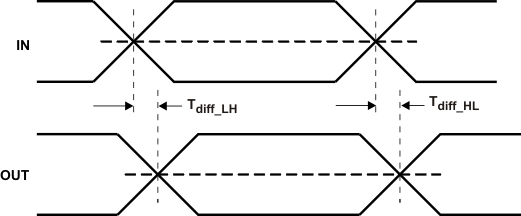Figure 1. Propagation Delay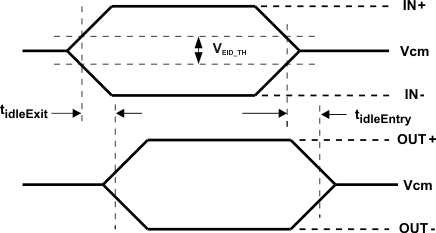Figure 2. Electrical Idle Mode Exit and Entry Delay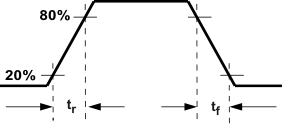Figure 3. Output Rise and Fall Times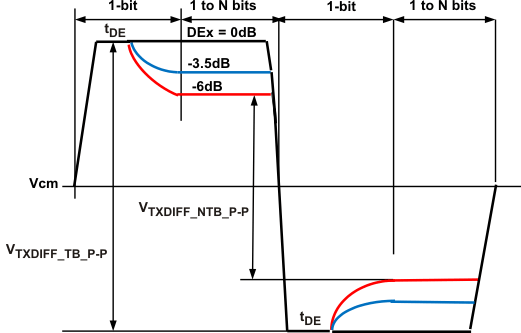Figure 4. Output De-Emphasis Levels OSx = NC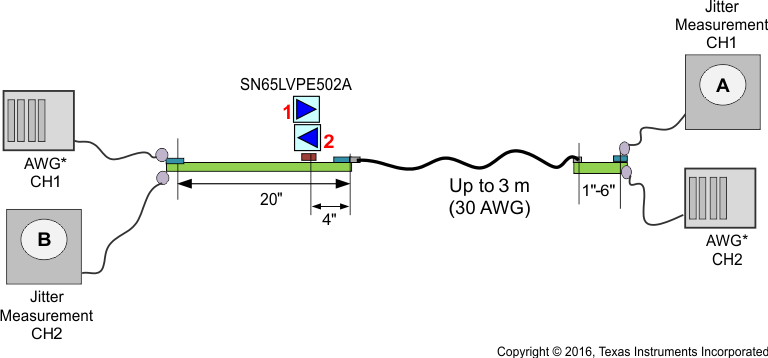Figure 5. Jitter Measurement Setup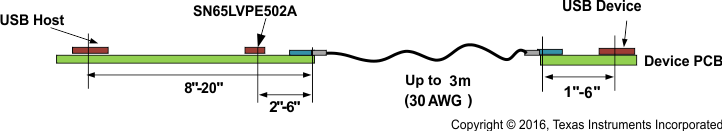For more detailed placement example of redriver, see Typical Characteristics.
Figure 6. Redriver Placement Example

### Table 1. Case I Fixed Output and Variable Input Trace (3-m Cable)

GRAPH TITLE FIGURE
DE = 0 dB, EQ = 0 dB, Input = 4 in., Output = 4 in., and 3-m Cable Figure 7
DE = 0 dB, EQ = 0 dB, Input = 8 in., Output = 4 in., and 3-m Cable Figure 8
DE = 0 dB, EQ = 0 dB, Input = 12 in., Output = 4 in., and 3-m Cable Figure 9
DE = 0 dB, EQ = 0 dB, Input = 16 in., Output = 4 in., and 3-m Cable Figure 10
DE = 0 dB, EQ = 0 dB, Input = 20 in., Output = 4 in., and 3-m Cable Figure 11
DE = 0 dB, EQ = 7 dB, Input = 24 in., Output = 4 in., and 3-m Cable Figure 12
DE = 0 dB, EQ = 7 dB, Input = 32 in., Output = 4 in., and 3-m Cable Figure 13
DE = 0 dB, EQ = 7 dB, Input = 36 in., Output = 4 in., and 3-m Cable Figure 14
DE = 0 dB, EQ = 15 dB, Input = 36 in., Output = 4 in., and 3-m Cable Figure 15
DE = 0 dB, EQ = 15 dB, Input = 48 in., Output = 4 in., and 3-m Cable Figure 16

### Table 2. Case II Fixed Input and Variable Output Trace (3-m Cable)

GRAPH TITLE FIGURE
DE = 0 dB, EQ = 7 dB, Input = 12 in., Output = 4 in., and 3-m Cable Figure 17
DE = 0 dB, EQ = 7 dB, Input = 12 in., Output = 8 in., and 3-m Cable Figure 18
DE = 0 dB, EQ = 7 dB, Input = 12 in., Output = 12 in., and 3-m Cable Figure 19
DE = 0 dB, EQ = 7 dB, Input = 12 in., Output = 16 in., and 3-m Cable Figure 20
DE = 0 dB, EQ = 7 dB, Input = 12 in., Output = 20 in., and 3-m Cable Figure 21

### Table 3. Case III Fixed Input and Variable Output Trace (No Cable)

GRAPH TITLE FIGURE
DE = 0 dB, EQ = 7 dB, Input = 12 in., and Output = 8 in. Figure 22
DE = 0 dB, EQ = 7 dB, Input = 12 in., and Output = 32 in. Figure 23
DE = 0 dB, EQ = 7 dB, Input = 12 in., and Output = 36 in. Figure 24
DE = –3.5 dB, EQ = 7 dB, Input = 12 in., and Output = 36 in. Figure 25
DE = –6 dB, EQ = 7 dB, Input = 12 in., and Output = 40 in. Figure 26
DE = –6 dB, EQ = 7 dB, Input = 12 in., and Output = 44 in. Figure 27

#### 6.7.1 Case I – Fixed Output, Variable Input Trace, and 3-m Cable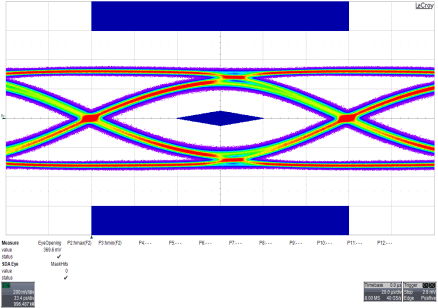Figure 7. DE = 0 dB, EQ = 0 dB, Input = 4 in.,
Output = 4 in., and 3-m CableFigure 9. DE = 0 dB, EQ = 0 dB, Input = 12 in.,
Output = 4 in., and 3-m Cable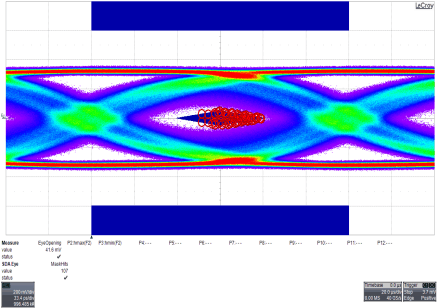Figure 11. DE = 0 dB, EQ = 0 dB, Input = 20 in.,
Output = 4 in., and 3-m Cable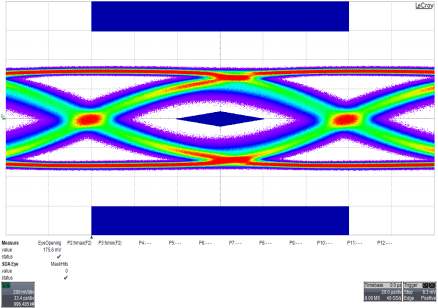Figure 13. DE = 0 dB, EQ = 7 dB, Input = 32 in.,
Output = 4 in., and 3-m Cable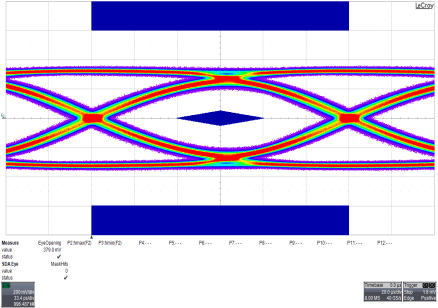Figure 15. DE = 0 dB, EQ = 15 dB, Input = 36 in.,
Output = 4 in., and 3-m Cable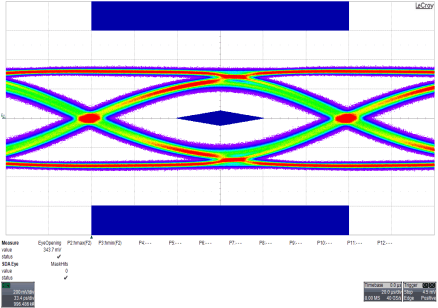Figure 8. DE = 0 dB, EQ = 0 dB, Input = 8 in.,
Output = 4 in., and 3-m Cable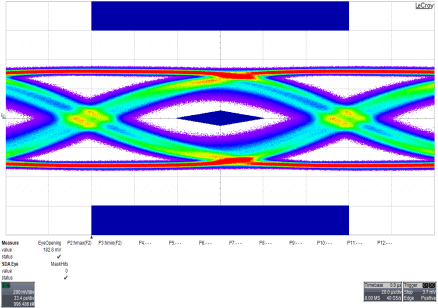Figure 10. DE = 0 dB, EQ = 0 dB, Input = 16 in.,
Output = 4 in., and 3-m Cable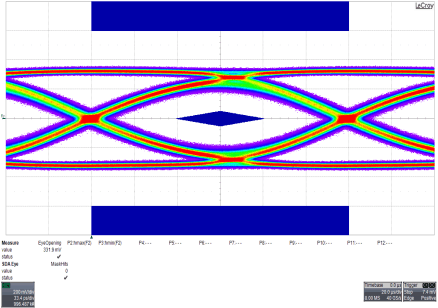Figure 12. DE = 0 dB, EQ = 7 dB, Input = 24 in.,
Output = 4 in., and 3-m Cable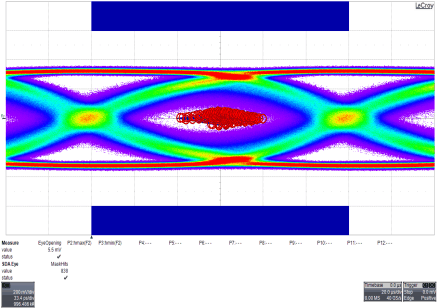Figure 14. DE = 0 dB, EQ = 7 dB, Input = 36 in.,
Output = 4 in., and 3-m Cable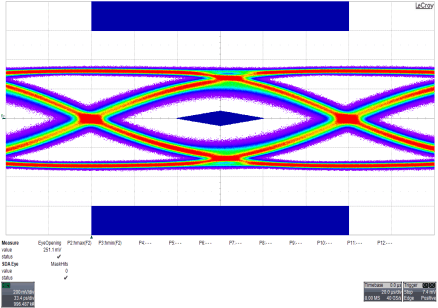Figure 16. DE = 0 dB, EQ = 15 dB, Input = 48 in.,
Output = 4 in., and 3-m Cable

#### 6.7.2 Case II – Fixed Input, Variable Output Trace, and 3-m CableFigure 17. DE = 0 dB, EQ = 7 dB, Input = 12 in.,
Output = 4 in., and 3-m Cable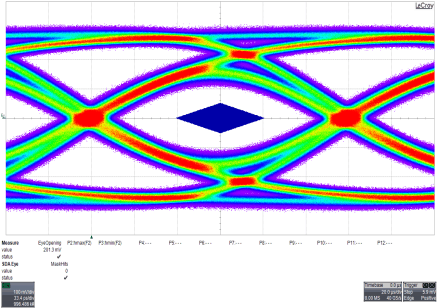Figure 19. DE = 0 dB, EQ = 7 dB, Input = 12 in.,
Output = 12 in., and 3-m Cable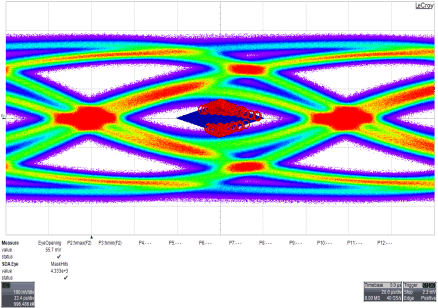Figure 21. DE = 0 dB, EQ = 7 dB, Input = 12 in., Output = 20 in., and 3-m CableFigure 18. DE = 0 dB, EQ = 7 dB, Input = 12 in.,
Output = 8 in., and 3-m CableFigure 20. DE = 0 dB, EQ = 7 dB, Input = 12 in.,
Output = 16 in., and 3-m Cable

#### 6.7.3 Case III – Fixed Input and Variable Output Trace (No Cable)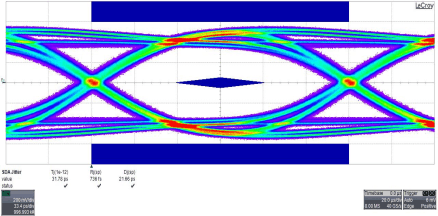Figure 22. DE = 0 dB, EQ = 7 dB, Input = 12 in.,
and Output = 8 in.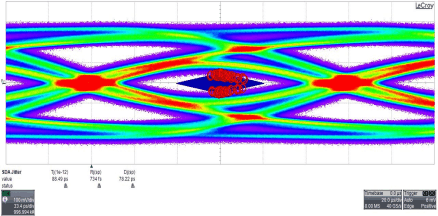Figure 24. DE = 0 dB, EQ = 7 dB, Input = 12 in.,
and Output = 36 in.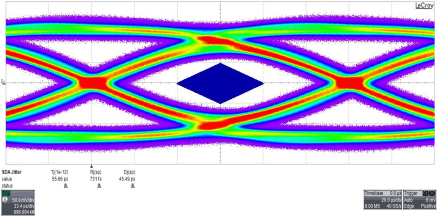Figure 26. DE = –6 dB, EQ = 7 dB, Input = 12 in.,
and Output = 40 in.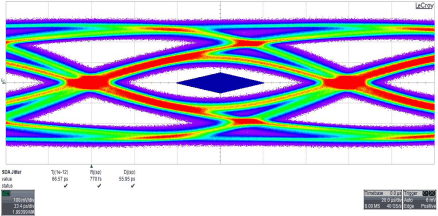Figure 23. DE = 0 dB, EQ = 7 dB, Input = 12 in.,
and Output = 32 in.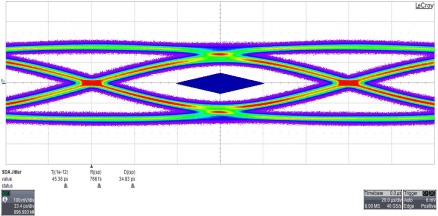Figure 25. DE = –3.5 dB, EQ = 7 dB, Input = 12 in.,
and Output = 36 in.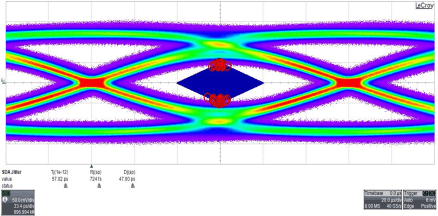Figure 27. DE = –6 dB, EQ = 7 dB, Input = 12 in.,
and Output = 44 in.